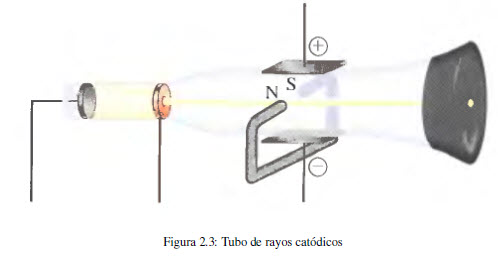Dalton in his atomic theory considers the atom as the basic unit of an element that can take part in a chemical reaction. For Dalton the atom was indivisible. However, work carried out after 1850 showed that atoms are made up of simpler particles, called subatomic particles.

The first evidence for atomic structure was provided in the early 1800s by the English chemist Humphry Davy (1778-1829). Davy found that electric current decomposed certain substances, suggesting that the elements of a compound were held together by electrical forces. In 1832 Michael Faraday (1791-1867) determined the amount of current needed to perform the electrolysis of a substance. In later studies together with George Stoney (1826-1911) they led Faraday to relate the unit of electric charge, which he called the electron, with the atom.

The first experimental evidence of the existence of electrons derives from studies carried out with the cathode ray tube. This device, used in television monitors, consists of a glass tube from which almost all the air has been evacuated and into which two plates (electrodes) connected to a high voltage source are inserted. It is observed that the negative electrode (cathode) emits an invisible beam that is directed towards the positive electrode (anode). Using a perforated positive electrode and placing a fluorescent screen behind it, it can be observed that the beam follows a straight path.
In later experiments, the cathode ray beam was subjected to electric and magnetic fields, observing deviations with respect to the rectilinear trajectory that implies the presence of negatively charged particles in said ray (electrons).In 1897 JJ Thomson (1856-1940) studied the change in the trajectory of cathode rays when subjected to simultaneous electric and magnetic fields, obtaining the relationship between the charge and the mass of the electron.

e/m = 1.75882 10 8 coulombs/gram

Once the relationship between mass and charge has been determined, it is necessary to design a new experiment that allows calculating one of the two magnitudes, obtaining the other from said relationship.

In 1909, Robert Millikan (1868-1953) carried out an experiment that determined the charge of the electron. Millikan's experiment consisted of spraying oil and studying the movement of fine droplets within an electric field. The drops were charged electrically by action of X-rays and by adjusting the voltage between the plates it is possible to compensate the gravitational force with the electrostatic force, at this point the drop levitates and the applied potential allows the calculation of the electric charge. This value is 1.60218 10 -19 coulombs. Since the charge/mass ratio is 1.75882 10 8 C/g, a simple conversion factor gives the mass of the electron.\begin{equation}
m=\frac{1\;g}{1.75882\;10^{8}\;C}\;1.60218\;10^{-19}\;C=9.10940\;10^{ -28}\;g
\end{equation}
The mass of the electron is 1836 times less than the mass of the hydrogen atom.